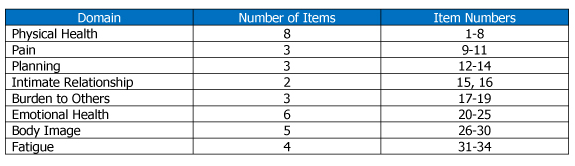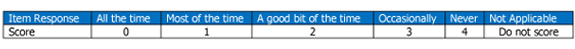# Instrument Scoring

The LupusQoL consists of 34 items in eight domains. The number of items in each domain and the item numbers that refer to that domain are tabulated below:The LupusQoL has a five-point Likert response scale as follow and the responses are scored as tabulated:Each domain of the LupusQoL is scored separately.

### Scoring Method:

1. Calculate the mean raw domain score by adding up the item response scores for each domain and dividing this total by the number of items in that domain.
2. Transform this mean raw domain score by dividing by four (the number of Likert responses [five] minus one) and then multiplying by 100, as below:

Mean raw domain score
------------------------------- x 100 = Transformed score for domain
4

Transformed scores range from 0 (worst HRQoL) to 100 (best HRQoL)

Transformed domain scores can be obtained when at least 50% of the items are answered. The mean raw domain score is then calculated by totaling the item response scores of the answered items and dividing by the number of answered items. A non-applicable response is treated as unanswered and the domain score calculated as above.

### Time to Complete:

Patients typically completed the LupusQoL in less than 10 minutes.

The scoring and the transformation of the scores takes approximately five minutes.